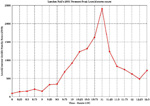custom search of Landon Noll's web sites

## What is calc?

[chongo's home] [Astronomy] [Mathematics] [Prime Numbers] [Programming] [Technology] [contacting Landon]

What is calc?
Calc is an interactive calculator which provides for easy large numeric calculations, but which also can be easily programmed for difficult or long calculations. It can accept a command line argument, in which case it executes that single command and exits. Otherwise, it enters interactive mode. In this mode, it accepts commands one at a time, processes them, and displays the answers. In the simplest case, commands are simply expressions which are evaluated. For example, the following line can be input:
```3 * (4 + 1)
```
and the calculator will print:
```15
```
Calc as the usual collection of arithmetic operators +, -, /, * as well as ^ (exponentiation), % (modulus) and // (integer divide). For example:
```3 * 19^43 - 1
```
will produce:
```29075426613099201338473141505176993450849249622191102976
```
Notice that calc values can be very large. For example:
```2^23209-1
```
will print:
```402874115778988778181873329071 ... many digits ... 3779264511
```
The special '.' symbol (called dot), represents the result of the last command expression, if any. This is of great use when a series of partial results are calculated, or when the output mode is changed and the last result needs to be redisplayed. For example, the above result can be modified by typing:
```. % (2^127-1)
```
and the calculator will print:
```39614081257132168796771975167
```
For more complex calculations, variables can be used to save the intermediate results. For example, the result of adding 7 to the previous result can be saved by typing:
```curds = 15
whey = 7 + 2*curds
```
Functions can be used in expressions. There are a great number of pre-defined functions. For example, the following will calculate the factorial of the value of 'old':
```fact(whey)
```
and the calculator prints:
```13763753091226345046315979581580902400000000
```
The calculator also knows about complex numbers, so that typing:
```(2+3i) * (4-3i)
cos(.)
```
will print:
```17+6i
-55.50474777265624667147+193.9265235748927986537i
```
The calculator can calculate transcendental functions, and accept and display numbers in real or exponential format. For example, typing:
```config("display", 70)
epsilon(1e-70)
sin(1)
```
prints:
```0.8414709848078965066525023216302989996225630607983710656727517099919104
```
Calc can output values in terms of fractions, octal or hexadecimal. For example:
```config("mode", "fraction"),
(17/19)^23
base(16),
(19/17)^29
```
will print:
```19967568900859523802559065713/257829627945307727248226067259
0x9201e65bdbb801eaf403f657efcf863/0x5cd2e2a01291ffd73bee6aa7dcf7d1
```
All numbers are represented as fractions with arbitrarily large numerators and denominators which are always reduced to lowest terms. Real or exponential format numbers can be input and are converted to the equivalent fraction. Hex, binary, or octal numbers can be input by using numbers with leading '0x', '0b' or '0' characters. Complex numbers can be input using a trailing 'i', as in '2+3i'. Strings and characters are input by using single or double quotes.

Commands are statements in a C-like language, where each input line is treated as the body of a procedure. Thus the command line can contain variable declarations, expressions, labels, conditional tests, and loops. Assignments to any variable name will automatically define that name as a global variable. The other important thing to know is that all non-assignment expressions which are evaluated are automatically printed. Thus, you can evaluate an expression's value by simply typing it in.

Many useful built-in mathematical functions are available. Use the:
```help builtin
```
command to list them.

You can also define your own functions by using the 'define' keyword, followed by a function declaration very similar to C.
```define f2(n)
{
local ans;

ans = 1;
while (n > 1)
ans *= (n -= 2);
return ans;
}
```
Thus the input:
```f2(79)
```
will produce;
```1009847364737869270905302433221592504062302663202724609375
```
Functions which only need to return a simple expression can be defined using an equals sign, as in the example:
```define sc(a,b) = a^3 + b^3
```
Thus the input:
```sc(31, 61)
```
will produce;
```256772
```
Variables in functions can be defined as either 'global', 'local', or 'static'. Global variables are common to all functions and the command line, whereas local variables are unique to each function level, and are destroyed when the function returns. Static variables are scoped within single input files, or within functions, and are never destroyed. Variables are not typed at definition time, but dynamically change as they are used.

```help overview